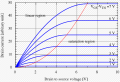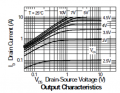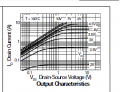# Mosfet as LED driver in feedback loop of opamp?

#### ridgerunnersjw

Joined Jan 6, 2021
5
Hello....
I have an n mosfet with a high side LED (between drain and 12V) and a 10 ohm from source to ground....The gate is driven by the output of an opamp which places the FET in the feedback loop. I am running this on LTSpice simulation. There is a resistor for gain tapping from the 10 ohm / source connection back to the negative input of the opamp. I am running a voltage into the positive terminal of the opamp and then monitoring Vgs, Vds and power (Vds * Is).

Somewhere along the way I have a misunderstanding.....Vds is > Vgs as seen on simulator....As an example I see Vds = 7V and Vgs is 2.5V (datasheet Vgs typ 1V , max 2.5V)...When I look at power it is a whopping 1.33W. So the questions:

Firstly Vds > Vgs means I should be in saturation mode correct?
If saturation I would have thought I could have used datasheet Rdson (0.18 ohms) and multiply by the 190mA (squared) current to get a reasonable power but when I take Vds / Id (using LTSPICE) I get Rdson of about 37 ohms. This seems to imply I am in the ohmic region . Can someone explain to me what I am missing here?

I don't seem to understand how Vds = 7V when Vgs = 2.5V and I am drawing about 190mA thru Id?

I really would like a good explanation that ties back into the Vds - Id characteristic curves for different Vgs because using that and comparing to what I see on LTSpice definitely isn't making sense.

Mosfet ZXMN3A01F

Thank you

#### Papabravo

Joined Feb 24, 2006
19,551
Unfortuneately, it seems you have some serious misunderstandings about how an enhancement mode MOSFET actually works. Let me break it down for you.
1. There is a silicon channel that connects the drain and the source.
2. The drain current can take values from 0 up to some maximum value.
3. The drain current is controlled by the voltage difference from the gate to the source.
4. There is a minimum threshold voltage between the gate and the source, below which the drain current is very small. This value of Vgs, where the channel between the drain and the source BEGINS to conduct, is called Vgs(th).
5. As Vgs continues to increase the resistance of the channel decreases until it reaches rds(on). Further increases in Vgs will not increase the drain current.
Missed point number 1: If there is a resistor between the source and GROUND, the voltage on the source will rise to the value of the voltage on the drain, reducing the value of Vgs. So in this configuration Vgs must be greater than Vd in order to turn the device on. It is the potential DIFFERENCE between Vg and Vs that turns the device on. the relationship between Vgs and Vds is NOT relevant.

In order to go further you need to present an actual schematic, so the explanations will make more snese.

#### crutschow

Joined Mar 14, 2008
31,089
If saturation I would have thought I could have used datasheet Rdson (0.18 ohms) and multiply by the 190mA (squared) current to get a reasonable power
You are apparently misunderstanding the saturation region of a MOSFET (it is not the same as for a BJT, which leads to a lot of confusion).

If Vds>Vgs then the transistor is indeed in the high linear or saturation (constant-current) region (near or to the right of the red line below).
But that means you are not in the bottom left of the linear region where the MOSFET is fully turned on and the value for Rds(on) applies (typically obtained for a Vgs of a volt or more above Vgs(th)).

If you had 7V across the Rds(on) value, then the transistor current would be 7V / 0.18Ω = 38.9A and the power dissipated would be 272W, which obviously you don't have.
The MOSFET power dissipated is 7V * 190mA = 1.33W as you measured.

37 ohms is just the calculated effective Rds under your particular operating conditions.

Make sense now?•Papabravo

#### ridgerunnersjw

Joined Jan 6, 2021
5
Unfortuneately, it seems you have some serious misunderstandings about how an enhancement mode MOSFET actually works. Let me break it down for you.
1. There is a silicon channel that connects the drain and the source.
2. The drain current can take values from 0 up to some maximum value.
3. The drain current is controlled by the voltage difference from the gate to the source.
4. There is a minimum threshold voltage between the gate and the source, below which the drain current is very small. This value of Vgs, where the channel between the drain and the source BEGINS to conduct, is called Vgs(th).
5. As Vgs continues to increase the resistance of the channel decreases until it reaches rds(on). Further increases in Vgs will not increase the drain current.
Missed point number 1: If there is a resistor between the source and GROUND, the voltage on the source will rise to the value of the voltage on the drain, reducing the value of Vgs. So in this configuration Vgs must be greater than Vd in order to turn the device on. It is the potential DIFFERENCE between Vg and Vs that turns the device on. the relationship between Vgs and Vds is NOT relevant.

In order to go further you need to present an actual schematic, so the explanations will make more snese.
Thank you for the responses....

The voltage on the source rises based on the Id current, it rises towards the drain . The drain is about 9V while the source is about 2V. The gate Vg was about 4.5V. So I am above the typ. 1V threshold and channel is NOT in cutoff. As you can see the potential difference that you mention is being met. My understanding as crutschow shows on the graph is that I am in saturation mode (to the right of the red line). But clearly as he points out I cannot be here because power would be thru the roof.

As I type all this I am beginning to wonder: Do the curves show what current the channel can support NOT necessarily what current is in the channel? So can I actually have a large Vds > Vgs AND only run a small current through it AND still be in saturation mode?

The question I have been pondering is if I have 2.5V between the Vg and Vs points of the graph AND I have Vd = 9V and Vs = 2V where do I lie along the curve to support what I am seeing in LTSpice that suggests the currents and power I listed above?
(The low current ie 180mA would seem to suggest I am way over on the curve within the linear region NOT the saturation region, yet here the Vds is low and nowhere near what I am measuring......In the other extreme if you believe the Vds number and follow the Vgs curve you would think I am running at some high current which I am not)Last edited by a moderator:

#### crutschow

Joined Mar 14, 2008
31,089
Do the curves show what current the channel can support NOT necessarily what current is in the channel? So can I actually have a large Vds > Vgs AND only run a small current through it AND still be in saturation mode?
No and yes. It depends upon Vgs.
Vds and Ids are very sensitive to the value of Vgs.
A small change in Vgs can cause a large change in Ids (and thus Vds).
(To measure Vgs in your simulation touch node g and press the left mouse button. Hold the button down, go to node s, release the button.)

The feedback in your circuit causes U1 to adjust Vg (and thus Vgs) to give the proper current and voltage in the circuit for the particular V2 voltage being applied.
You just have to follow the curve (from MOSFET data sheet below) that gives you the Vds and Vgs voltages, as well as the Ids
current you are seeing.

But note that the Vgs for a specific current varies significantly from unit-to-unit, so what you are seeing may be somewhat different than what the typical graph shows.
Also, incremental changes of Vgs give an infinite number of curves between those shown so you will likely not find an exact point on the curves to fit your particular operating point. You have to extrapolate between the curves for that.
Alternately you can simulate the MOSFET you are using, with the DC Sweep function, to get the exact characteristic curves for that device.#### Audioguru again

Joined Oct 21, 2019
5,404
The graph in post #3 is not for your Mosfet
The datasheet for the Mosfet you are using shows a threshold voltage range as high as 2.5V for some of that Mosfet when the drain-source current is almost nothing at 0.25mA. It conducts a minimum of 2A when the Vgs is 4.5V but then the Mosfet will melt.

#### ridgerunnersjw

Joined Jan 6, 2021
5
No and yes. It depends upon Vgs.
Vds and Ids are very sensitive to the value of Vgs.
A small change in Vgs can cause a large change in Ids (and thus Vds).
(To measure Vgs in your simulation touch node g and press the left mouse button. Hold the button down, go to node s, release the button.)

The feedback in your circuit causes U1 to adjust Vg (and thus Vgs) to give the proper current and voltage in the circuit for the particular V2 voltage being applied.
You just have to follow the curve (from MOSFET data sheet below) that gives you the Vds and Vgs voltages, as well as the Ids
current you are seeing.

But note that the Vgs for a specific current varies significantly from unit-to-unit, so what you are seeing may be somewhat different than what the typical graph shows.
Also, incremental changes of Vgs give an infinite number of curves between those shown so you will likely not find an exact point on the curves to fit your particular operating point. You have to extrapolate between the curves for that.
Alternately you can simulate the MOSFET you are using, with the DC Sweep function, to get the exact characteristic curves for that device.
View attachment 258270
Thank you ....this is a really good response! Using the graph below (I know this is at 150C but the 25C graph seems to show only 100mA max is supported). I did exactly what you are saying in spice....Now I know from my simulator Vgs = 2.5V, Vds = 7V and Id = 190mA. If I use the graph below and go onto the 2.5V curve and move over to 190mA (roughly the 200mA line), I get a Vds ~ 133mV. If I take the other route and use the Vgs = 2.5V curve and go to Vds = 7V I get a current of 500mA.....Neither of these come anywhere close to representing what I see which is 190mA and Vds = 7V#### Audioguru again

Joined Oct 21, 2019
5,404
A Mosfet graph usually shows one with a "typical" (middle of the range) sensitivity. But a real Mosfet can have high sensitivity or have low sensitivity.
Its threshold Vgs voltage gives a hint to its amount of sensitivity.

#### crutschow

Joined Mar 14, 2008
31,089
I know this is at 150C but the 25C graph seems to show only 100mA max is supported)
What?
The 25C graph in post #5 goes to 10A.

And you can't use a 150C graph and expect it to correlate with your 25C simulation.

I believe I've done as much as I can to explain what's going on.
As I've stated, you are not going to find exact correlation between the graph and your simulation.
Don't know what else to say so I'm not.

#### RPLaJeunesse

Joined Jul 29, 2018
222
My suggestions: 1) Ignore the graphs, 2) Ignore Vgs, 3) Just think about how the op-amp will drive Vgs such that its inputs will match, thus making the drain be 2V and the 10 Ohm resistor pass 200mA (and 0.4W dissipation). OK, now you have no gate current, so that 200 ma flows through the transistor and LED. Subtract 2V and the LED forward voltage from the supply, the result is what will be the FET Vds. Now that 7.2V number seems reasonable, and the transistor will dissipate 7.2*0.2 = 1.44W. Oh, and don't forget the lag in feedback due to FET capacitance. If the op-amp is fast enough you will have a nice power oscillator!

#### CapnAl

Joined Jun 10, 2020
2
Thank you for the responses....

The voltage on the source rises based on the Id current, it rises towards the drain . The drain is about 9V while the source is about 2V. The gate Vg was about 4.5V. So I am above the typ. 1V threshold and channel is NOT in cutoff. As you can see the potential difference that you mention is being met. My understanding as crutschow shows on the graph is that I am in saturation mode (to the right of the red line). But clearly as he points out I cannot be here because power would be thru the roof.

As I type all this I am beginning to wonder: Do the curves show what current the channel can support NOT necessarily what current is in the channel? So can I actually have a large Vds > Vgs AND only run a small current through it AND still be in saturation mode?

The question I have been pondering is if I have 2.5V between the Vg and Vs points of the graph AND I have Vd = 9V and Vs = 2V where do I lie along the curve to support what I am seeing in LTSpice that suggests the currents and power I listed above?
(The low current ie 180mA would seem to suggest I am way over on the curve within the linear region NOT the saturation region, yet here the Vds is low and nowhere near what I am measuring......In the other extreme if you believe the Vds number and follow the Vgs curve you would think I am running at some high current which I am not)

View attachment 258256
What it seems that everyone is missing, is that the drop across an LED of moderate power is going to be ~3V. So naturally the drain voltage of the MOSFET is going to be 9V. (12-3 = 9.) For the circuit to maintain a constant current thru the LED, the MOSFET must drop any excess voltage. No matter what the "curves" say. It HAS TO operate in the linear region - that's the nature of an analog regulator, whether voltage or current. ;-)

#### RPLaJeunesse

Joined Jul 29, 2018
222
What it seems that everyone is missing, is that the drop across an LED of moderate power is going to be ~3V. So naturally the drain voltage of the MOSFET is going to be 9V. (12-3 = 9.) For the circuit to maintain a constant current thru the LED, the MOSFET must drop any excess voltage. No matter what the "curves" say. It HAS TO operate in the linear region - that's the nature of an analog regulator, whether voltage or current. ;-)
Agreed, but you missed that the simulation will force 2 V across the current sense resistor, thus giving Vds=~7V as the OP stated originally.

#### CapnAl

Joined Jun 10, 2020
2
Agreed, but you missed that the simulation will force 2 V across the current sense resistor, thus giving Vds=~7V as the OP stated originally.
Didn't miss it; that's part of what happens in a closed-loop LED current regulator. 2V into U1 will naturally put 2V across the sense resistor (R1) in that circuit. Everybody else seems focused on why there's 7V across the MOSFET using data sheet curves etc., totally neglecting the fact that there HAS to be. I just stated WHY from a totally different viewpoint. (Technician vs. engineer. ;-) )